Popular tags: Support, Resistance, Trend, Range, Fibonacci, Wave, Divergence, Multi time frames(MTF), Swing, Volume, Overbought, Oversold

Featured

Availability: In Stock
Price: \$199.00USD \$120.00USD

## Available Options

Qty:     - OR -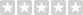(0/5)  0 reviews  |  Write a review

# Please note that this purchase is a 1-Year license. About License renew.ABCD 4-point chart pattern is a very popular and well known pattern in chart technical analysis, it consists of 4 points and 3 legs. It exhibits three continued lines in a specific trend. The concept is to compare the retracement level between legs. For example, when leg BC makes a 0.382 or 38.2% Fibonacci retracement of the BA leg or AB, it means that the price difference from point C to B is equal to 38.2% of the price difference from point B to A.

Please note that this indicator is only for ABCD 4-point chart pattern in uptrend, in particular, it must be an uptrend from point A to point B as shown in picture below.

# Here is the formula to calculate it: |Price of C - Price of D| / |Price of A - Price of B|. Also, the price difference between B to C and A to B must reach a given ratio, BC:AB calculation is the same as AB:CD. Point B must be higher than point A in a given percentage.

## How it works?

The indicator will automatically detect the ABCD 4-point chart pattern based on the input values we use. It will draw three lines to connect point A, B, C and D once all four points satisfy the condition. It will also display the letter of A, B, C and D above or below in each corresponding bar. The indicator draws seven extension lines from point D as the support and resistance levels. The default levels are based on the Golden Ratio. You can change the ratios from input. The indicator will start to plot the lines as soon as the pattern is confirmed, the trend may continue to go up after the event.

It shows the Fibonacci retracement support and resistance levels for a given range and displays the corresponding ratio on the right side of the levels. The price range and levels are adjustable by user, you can easily identify the major and minor support/resistance levels from the chart with your desired inputs.

Features and Inputs:

No repaint, all drawing objects show up immediately right after the point D is detected.
Able to show patterns found in history data.
There is a Built-in alert function to easily set up an alert.
Works on all timeframes, including second, minute, hour, day, week, month.
Works on Renko, Heikin Ashi, Range, bar, candle chart types.
• Strength, this input is used to define the minimum number of bars to the left and right side of the swing point. For more details about this input, please check: https://goo.gl/sWBH7k
• B:A Ratio Min in %, this is the minimum price difference in percentage between point B and point A. If we enter 0.3 for this input, the indicator will check if B(swing high) was 0.3% higher than A(swing low).
• B:A Ratio Max in %, this is the maximum price difference in percentage between point B and point A. If we enter 2.5 for this input, the indicator will check if the price difference between point B and point A was less than 2.5%.
• BC:AB Ratio Min in %, this is the minimum ratio in percentage between section BC and AB. Here is the formula to calculate it: |Price of C - Price of B| / |Price of A - Price of B|.
• BC:AB Ratio Max in %, this is the maximum ratio in percentage between section BC and AB. Here is the formula to calculate it: |Price of C - Price of B| / |Price of A - Price of B|.
• CD:AB Ratio Min in %, this is the minimum ratio in percentage between section CD and AB. Here is the formula to calculate it: |Price of C - Price of D| / |Price of A - Price of B|.
• CD:AB Ratio Max in %, this is the maximum ratio in percentage between section CD and AB. Here is the formula to calculate it: |Price of C - Price of D| / |Price of A - Price of B|.
• Extension Range, this is the number of bars extend to the right side of the support and resistance lines, set it to 0 if you want to disable it.
• ABCD letter COLOR, the color of the letter for point A, B, C and D. It's very useful when we use more than one instance of this indicator on the same chart with different input sets. We can easily distinguish them by different letter color.
• ABCD Line COLOR, the color of the lines that connect point A, B, C and D. Similar functionality as the letter color.
• Show Fibo Level labels, enable this input will show up the "0%" and "100%" as label on corresponding Fibonacci levels.
• Level 1 to 5, are the support and resistance levels in percentage, please use negative value if you need a level below 0%, such as -61.8 means 61.8% below level 0%. The level 0% is the price level at Point A, and the level 100% is the price level at Point D, these two levels are fixed once the pattern formed.
• You can change the color of each level line from the following colors: silver, gray, white, maroon, red, purple, fuchsia, green, lime, olive, yellow, navy, blue, teal, aqua and orange.
• Line style: you can choose from: Solid, Dotted and Dashed.
1 is Solid like this: ______________________
2 is Dashed - - - - - - - - - - - - - - - - - - -
3 is dotted ............................................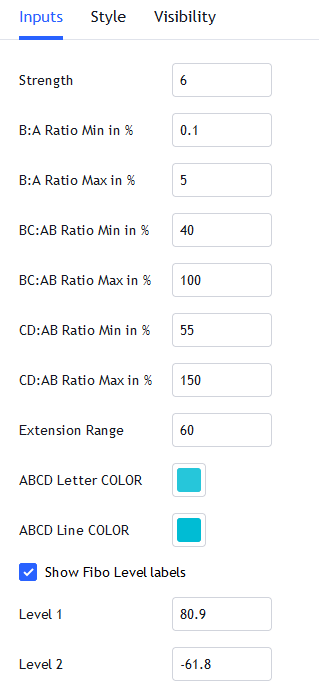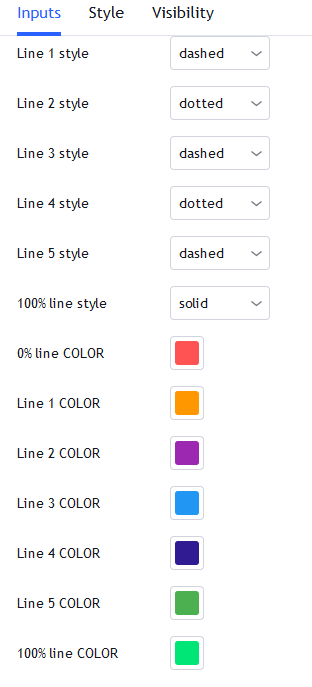It's very easy to set up an alert, just follow the 2 steps below.
1. Move your mouse pointer to the right end of the indicator name. Then click the three dots, as "step1" in the picture below.
2. Click the only option as "step2" in the picture below.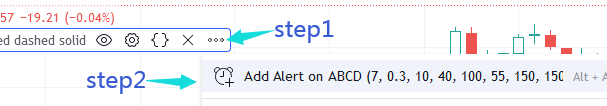You can also add the alert from top menu strip. Make sure you select the Condition as shown in pic below: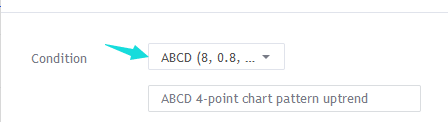You will get an alert message popup on the chart when there is an ABCD trend chart pattern detected.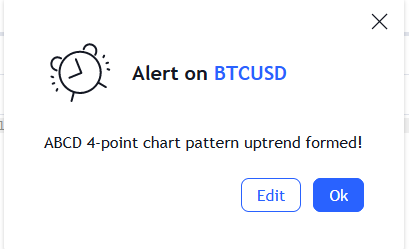Sometimes, we may need to focus on a particular pattern, which may require strict ratio parameters.
If we only want to detect an ABCD pattern, which qualify the following rules:
1. There must be at least 10 bars on both left and right sides of point A, B and C.
2. The price must raise around 0.8% from point A to point B
3. The ratio BC:AB must be around 70%
4. CD must have similar price difference of AB, in other words, price difference between point C and D should be similar to price difference between point A and B.

Then we can use the following inputs to detect such a pattern:
We need to enter 10 for the strength input value.
The price must raise around 0.8% from point A. We can use 0.75 for the min ratio of B:A and 0.85 for the max ratio of B:A, since 0.8 is between of 0.75 and 0.85. The value is in percentage, so 0.05 is only 0.05%, which is a very small amount of price change.
We should not simply use 100% for CD:AB ratio. The best way is to give a range from the min to the max percentage, since in real life we can hardly find any pattern has exact 100% as the CD:AB ratio.
Most of the time, the CD:AB ratio could be near 100%, such as 96%, 99% or 102%, so using 95% to 105% is a relatively reasonable range.

The picture below shows the indicator with the inputs we mentioned above, and the detected ABCD pattern.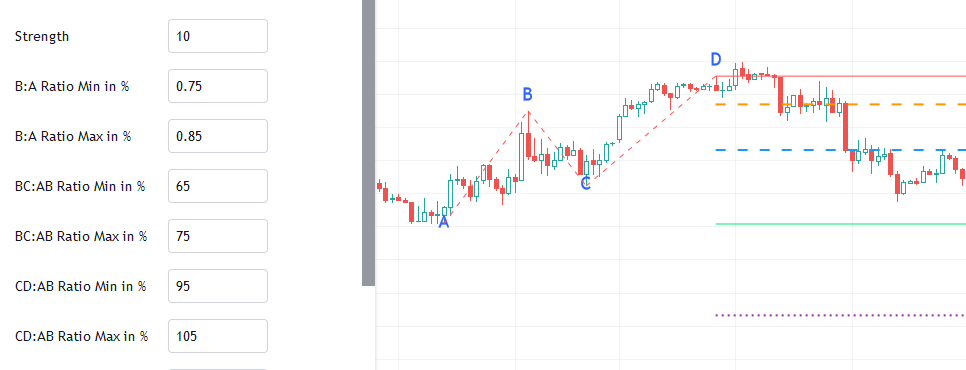Please note that, only the latest found pattern will show the Fibonacci levels. The patterns found in history will only show the lines that connect the A, B, C and D points and the letters, as shown in the pic below.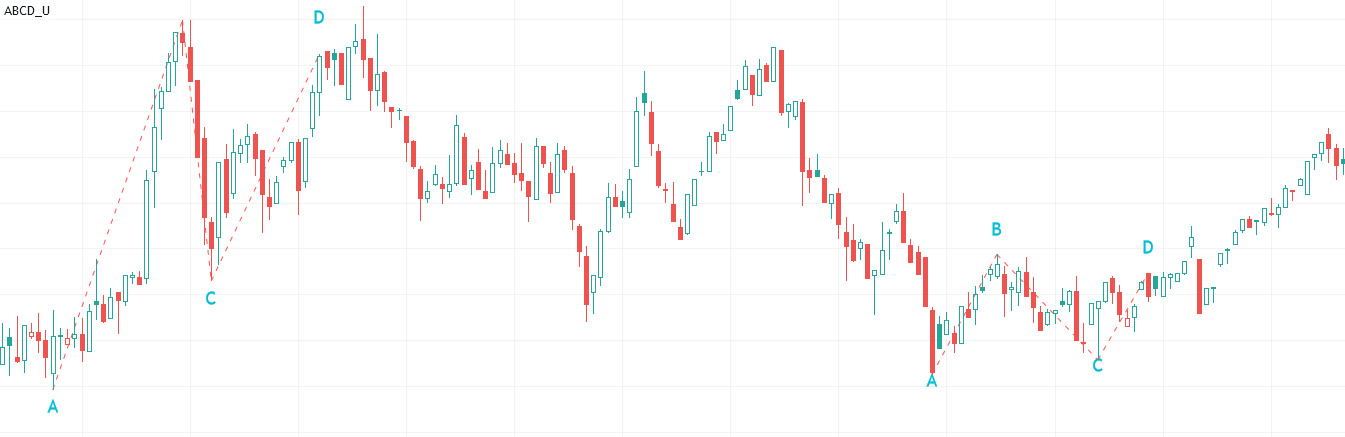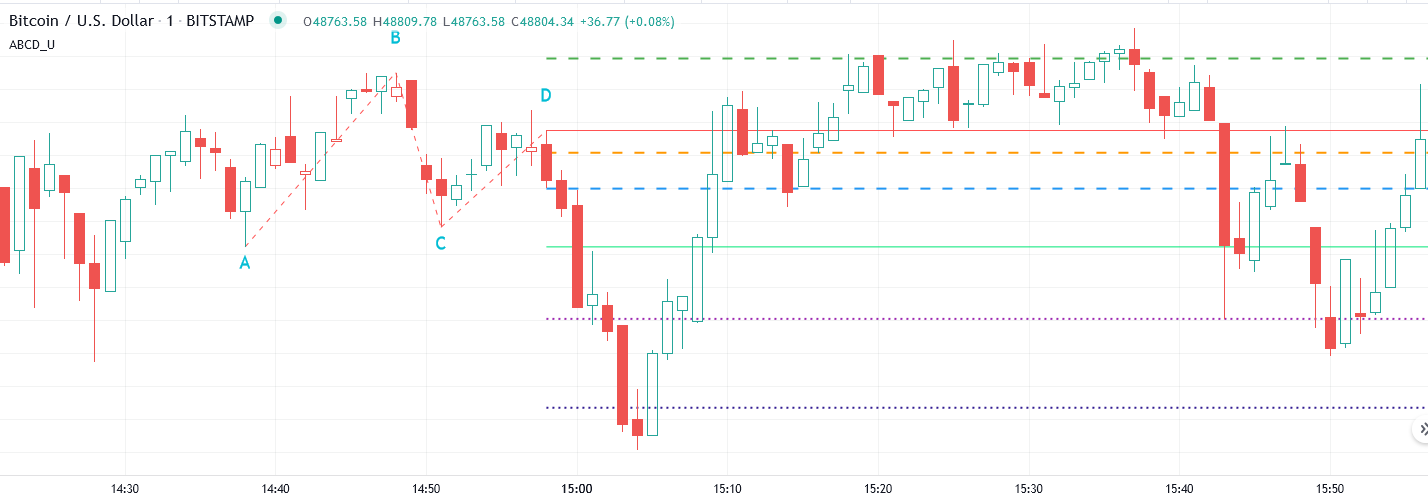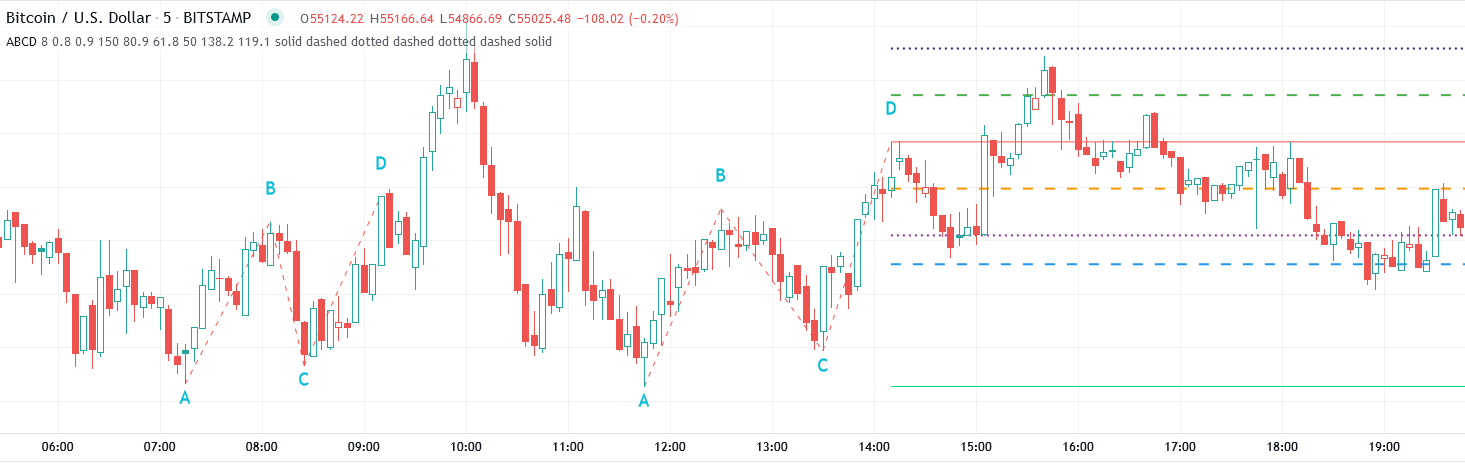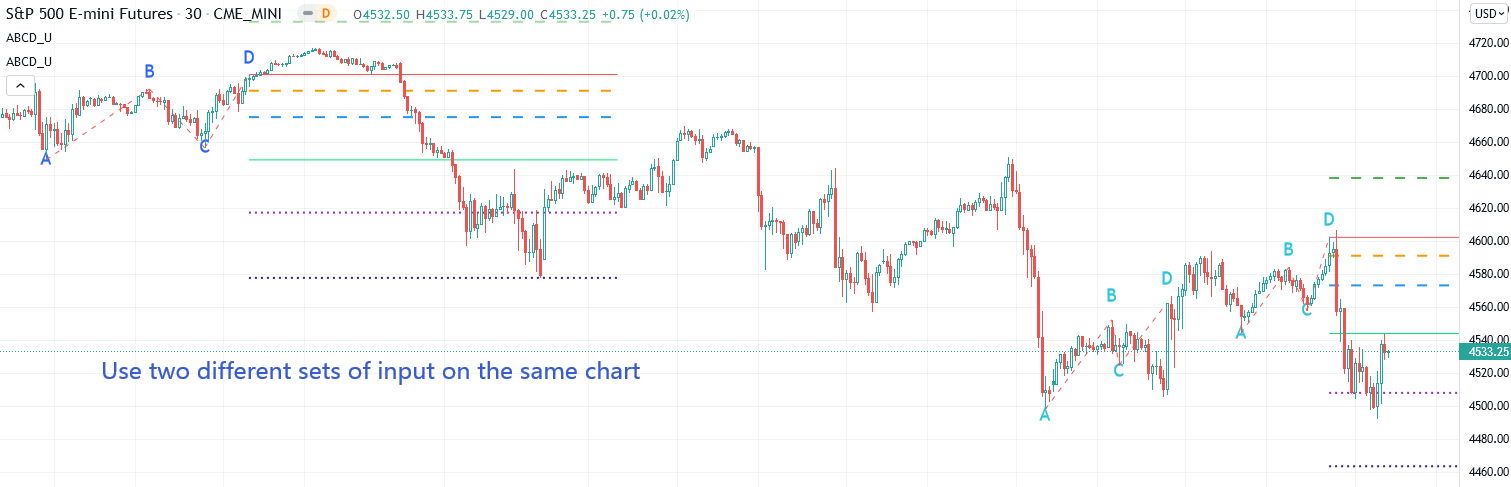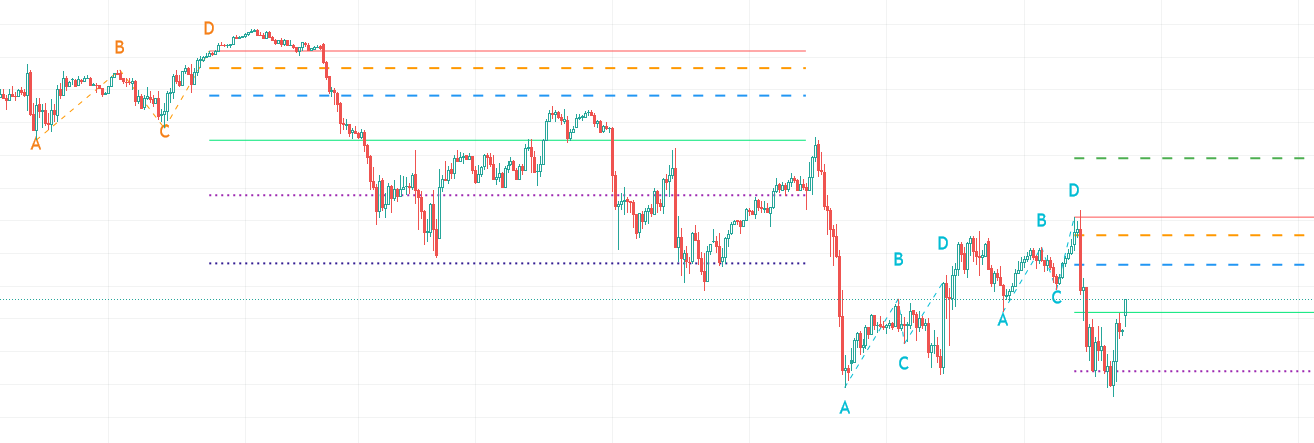Example of using the indicator on Heikin Ashi chart.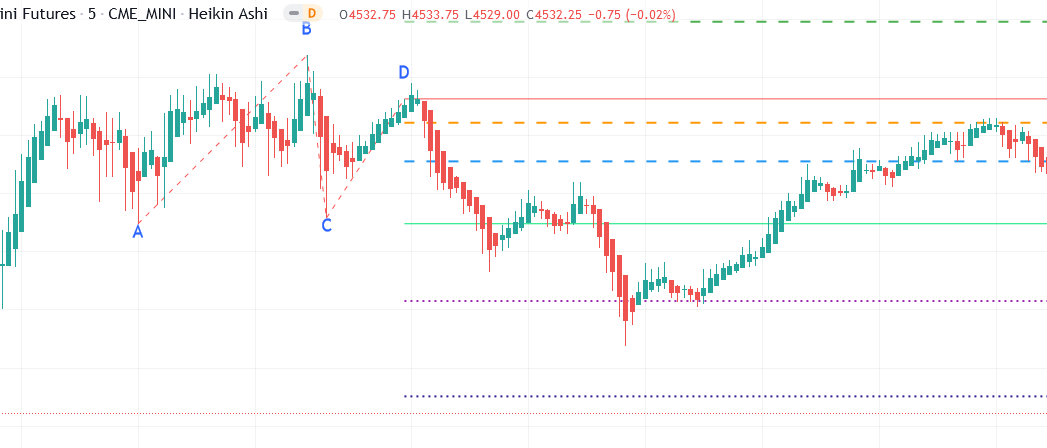Example of using the indicator on Renko chart.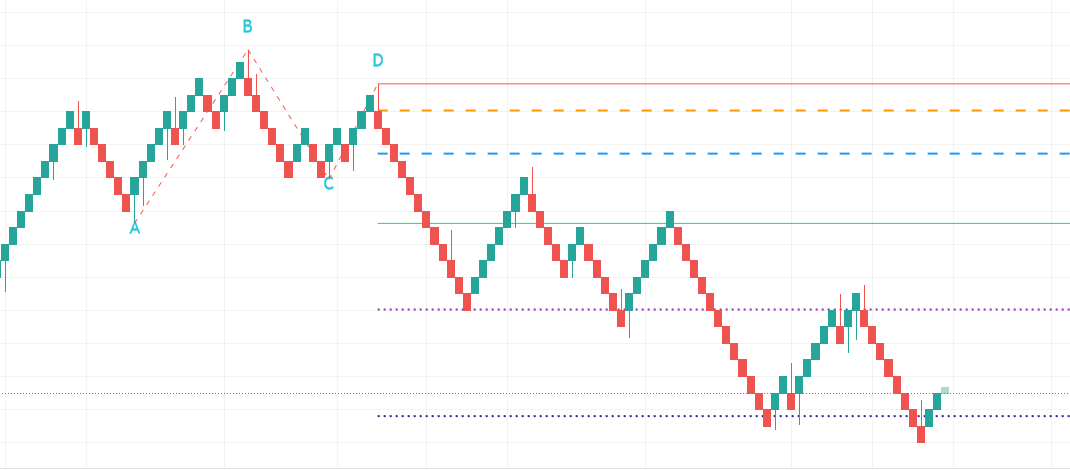## Write a review

Your Review: Note: HTML is not translated!

Enter the code in the box below:Latest
Welcome to Patternsmart
Converting your indicator from one platform to another.